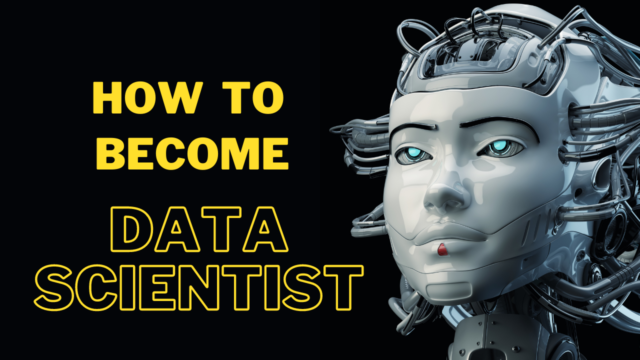Home How To How to Become a Data Scientist

# How to Become a Data Scientist

0Becoming a data scientist typically requires a combination of education, skills development, and practical experience. Here are some steps you can take to pursue a career in data science:

1. Education:

• Obtain a Bachelor’s degree: A degree in a quantitative field such as computer science, mathematics, statistics, physics, or engineering provides a strong foundation for data science.
• Pursue a Master’s degree: Although not always necessary, a Master’s degree in data science, computer science, or a related field can enhance your knowledge and credibility in the field.

2. Develop a strong foundation in mathematics and statistics:

• Data science relies heavily on mathematical and statistical concepts. Familiarize yourself with topics like linear algebra, calculus, probability, and statistical inference.

3. Learn programming languages:

• Python and R are widely used in data science. Familiarize yourself with these languages and learn libraries and frameworks commonly used for data analysis and machine learning, such as NumPy, pandas, scikit-learn, and TensorFlow.

4. Gain expertise in data manipulation and analysis:

• Learn how to collect, clean, and preprocess data. Familiarize yourself with SQL for database querying. Develop skills in data visualization to effectively communicate insights.

5. Understand machine learning and statistical modeling:

• Study machine learning algorithms, such as regression, classification, clustering, and dimensionality reduction. Learn about model evaluation, cross-validation, and hyperparameter tuning.

6. Practice with real-world projects:

• Undertake data science projects to gain hands-on experience. Work on datasets, solve problems, and apply the concepts and techniques you’ve learned. Build a portfolio showcasing your projects.